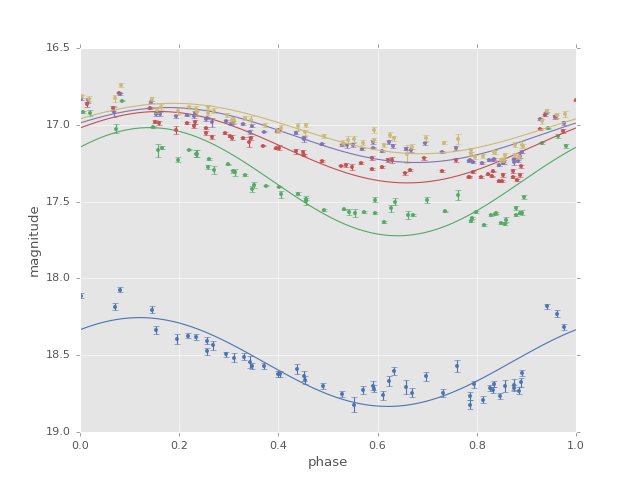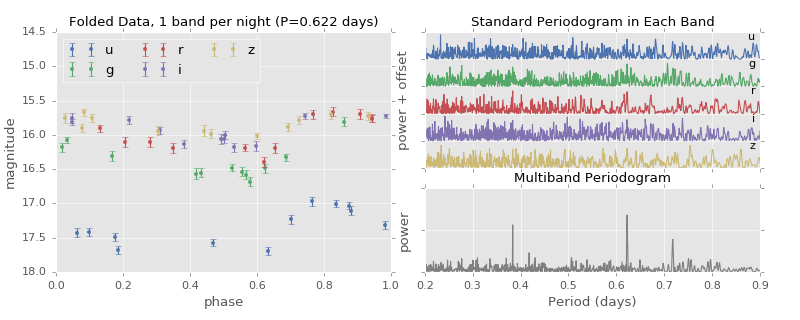# Multiband Lomb-Scargle Periodogram¶

Though classical periodogram approaches only handle a single band of data, multiband extensions have been recently proposed. `gatspy` implements one which was suggested by VanderPlas et al. The interface is almost identical to that discussed in Lomb-Scargle Periodogram, with the exception of the `fit()` method and `predict()` method requiring a specification of the filters.

Two versions of the multiband periodogram are available:

`LombScargleMultiband`
This class implements the flexible multiband model described in VanderPlas (2015). In particular, it uses regularization to push common variation into a base model, which effectively simplifies the overall model and leads to less background signal in the periodogram.
`LombScargleMultibandFast`
This class is a faster version of the multiband periodogram without regularization. This means that it cannot fit the same range of models as `LombScargleMultiband`, but essentially just combines several independent band-by-band fits.

Here is a quick example of finding the best period in multiband data. We’ll use `LombScargleMultibandFast` here. We start by loading the lightcurve (for more information, see Datasets (gatspy.datasets)):

```In : from gatspy import datasets, periodic

In : rrlyrae = datasets.fetch_rrlyrae()

In : lcid = rrlyrae.ids

In : t, mag, dmag, filts = rrlyrae.get_lightcurve(lcid)
```

With this lightcurve specified, we can now build and fit the model:

```In : model = periodic.LombScargleMultibandFast(fit_period=True)

In : model.optimizer.period_range=(0.5, 0.7)

In : model.fit(t, mag, dmag, filts);
```

And, as with the single-band version, the best period is now stored as an attribute of the model:

```In : model.best_period
Out: 0.61431670719850195
```

Once the model is fit, we can then use the `predict()` method to look at the model prediction for any given band:

```In : tfit = np.linspace(0, model.best_period, 1000)

In : magfit = model.predict(tfit, filts='g')

In : magfit[:4]
Out: array([ 17.1411512 ,  17.13947457,  17.13780707,  17.13614876])
```

Below is a plot of the magnitudes at this best-fit period:We see that the simplest multiband model is just a set of offset sine fits to the individual bands. As in the single-band case, the model is not a particularly good fit to the data, but nevertheless it is useful in determining the period from the data.

A more sophisticated multiband approach involves model simplification via a regularization term that pushes common variation into a “base model”; this is slightly slower to compute, but can be accomplished with the `LombScargleMultiband` model. For example, here is a comparison of the single-band periodograms to this regularized multiband model on six months of sparsely-sampled LSST-style data:Notice in this figure that periodograms built from individual bands fail to locate the frequency, while the periodogram built from the entire dataset has a strong spike in power at the correct frequency.

For more information on these multiband methods, see the VanderPlas et al. paper, and the associated figure code at http://github.com/jakevdp/multiband_LS/